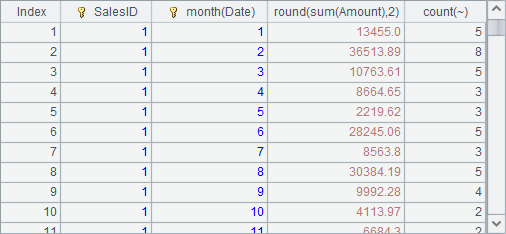# 序表是有结构的序列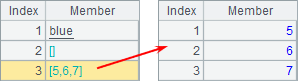A 1 =file("Order_Books.txt").import@t() 2 =demo.query("select * from CITIES") 3 =create(OrderID,Client,SellerId,Amount,OrderDate)

A1从文本文件生成序表，获得的结果就是上面的序表。A2通过SQL生成序表，A3指定字段名创建空序表。A2A3中的数据如下：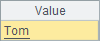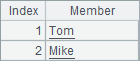A 1 =file("Order_Books.txt").import@t() 2 =A1.select(Amount>=20000 && month(Date)==5) 3 =A1.sort(SalesID,-Date) 4 =A1.groups(SalesID, month(Date); round(sum(Amount),2), count(~))

A2中查询出Amount字段大于等于20000Date5月的记录：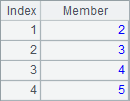A3将记录按照SalesID字段升序排列，SalesID一样时将按Date降序排列：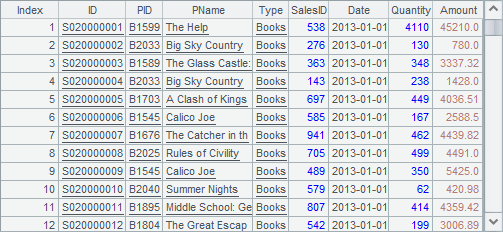A4SalesID和月份，对每一组数据的Amount求和，并计算该组订单计数：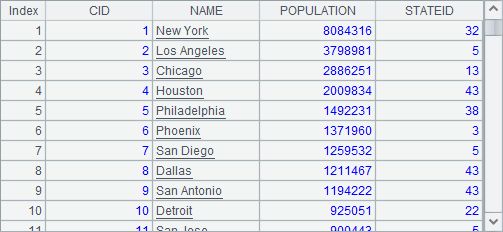A 1 =file("Order_Books.txt").import@t() 2 =A1.groups(month(Date); sum(decimal(string(Amount))):TotalAmount, count(~):Count) 3 =A2.derive(round(TotalAmount/TotalAmount[-1]-1,4): compValue)

A2中先统计出每月的总销售额：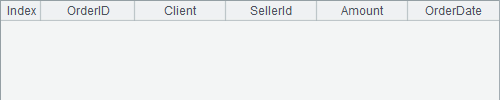A3中计算出最终结果如下：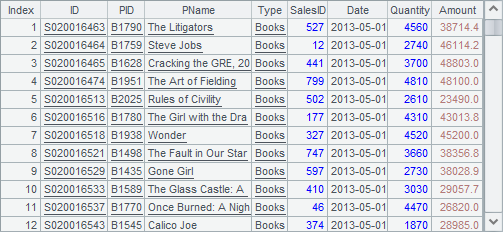A B 1 =file("Order_Books.txt").import@t() 2 Big =A1.select(Quantity>1000) 3 Importance =A1.select(Amount>10000) 4 =B2^B3 =A1\A4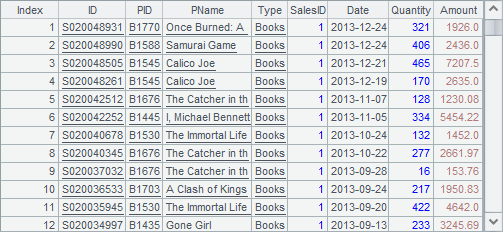B4中，用全部的订单与问题①的结果做差集，即可得到问题②的答案：